# Redlich Kwong EOS in FPROPS

Work on this continues as part of GSoC 2011 (Richard Towers). See also FPROPS, PengRobinson EOS in FPROPS.

## Overview

The Redlich-Kwong equation of state is one of several cubic equations of state that FPROPS aims to implement in the future. In its basic form it relates pressure to temperature and relative volume: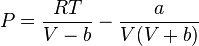\begin{align} P=\frac{RT}{V-b}-\frac{a}{V(V+b)} \end{align}

## Departure Functions

Other thermodynamic properties can be calculated from the departure functions:

Helmholtz Energy:\begin{align} A-A^{0}=-RT\ln\frac{V-b}{V}-\frac{a}{b}\ln\frac{V+b}{V}-RT\ln\frac{V}{V^{0}} \end{align}

Entropy: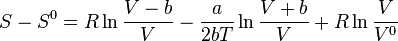\begin{align} S-S^{0}=R\ln\frac{V-b}{V}-\frac{a}{2bT}\ln\frac{V+b}{V}+R\ln\frac{V}{V^{0}} \end{align}

Enthalpy: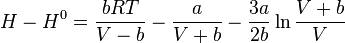\begin{align} H-H^{0}=\frac{bRT}{V-b}-\frac{a}{V+b}-\frac{3a}{2b}\ln\frac{V+b}{V} \end{align}

Internal Energy: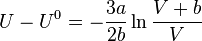\begin{align} U-U^{0}=-\frac{3a}{2b}\ln\frac{V+b}{V} \end{align}

Gibbs free energy:\begin{align} G-G^{0}=\frac{bRT}{V-b}-\frac{a}{V+b}-RT\ln\frac{V-b}{V}-\frac{a}{b}\ln\frac{V+b}{V}-RT\ln\frac{V}{V^{0}} \end{align}

Specific Heat Capacity at Constant Pressure:

Specific Heat Capacity at Constant Temperature:

## Other Properties

Fugacity: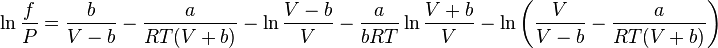\begin{align} \ln\frac{f}{P}=\frac{b}{V-b}-\frac{a}{RT(V+b)}-\ln\frac{V-b}{V}-\frac{a}{bRT}\ln\frac{V+b}{V}-\ln\left(\frac{V}{V-b}-\frac{a}{RT(V+b)}\right) \end{align}